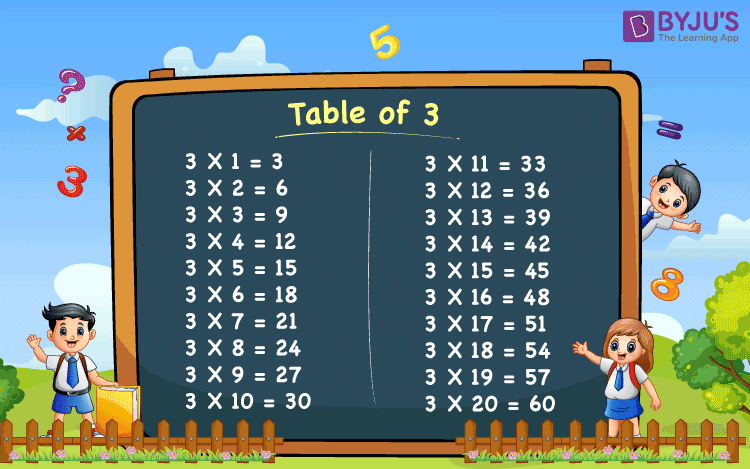# Table of 3

Table of 3 is provided here. It is essential for the students to memorise the table which will be beneficial in fast calculation. Below, we have provided the table for 3 which will be beneficial in time-based examination.

## Table of 3 Chart## Multiplication Table of 3

 3 x 1 = 3 3 x 2 = 6 3 x 3 = 9 3 x 4 = 12 3 x 5 = 15 3 x 6 = 18 3 x 7 = 21 3 x 8 = 24 3 x 9 = 27 3 x 10 = 30 3 x 11 = 33 3 x 12 = 36 3 x 13 = 39 3 x 14 = 42 3 x 15 = 45 3 x 16 = 48 3 x 17 = 51 3 x 18 = 54 3 x 19 = 57 3 x 20 = 60

Get More Maths Tables:-

Test your Knowledge on Table of 3